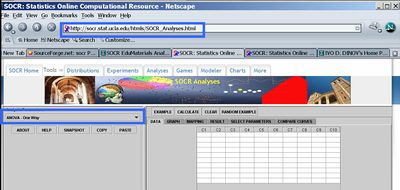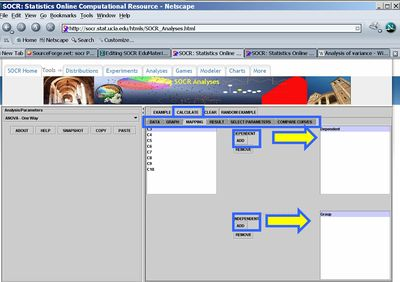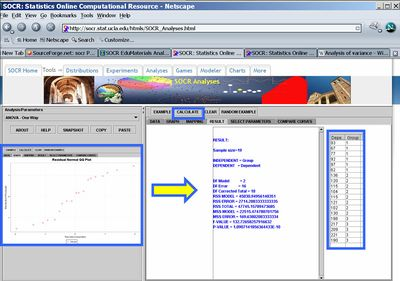# SOCR EduMaterials AnalysisActivities ANOVA 1

## This SOCR Activity demonstrates the utilization of the SOCR Analyses package for statistical Computing. In particular, it shows how to use Analysis of Variance (ANOVA) and how to interpret the results

• ANOVA Background: Analysis of variance (ANOVA) is a class of statistical analysis models and procedures, which compare means by splitting the overall observed variance into different parts. The initial techniques of the analysis of variance were pioneered by the statistician and geneticist R. A. Fisher in the 1920s and 1930s, and is sometimes known as Fisher's ANOVA or Fisher's analysis of variance, due to the use of Fisher's F-distribution as part of the test of statistical significance. Read more about ANOVA.
• SOCR ANOVA: Go to SOCR Analyses and select One-way ANOVA from the drop-down list of SOCR analyses, in the left panel. There are three ways to enter data in the SOCR ANOVA applet
• Click on the Example button on the top of the right panel.
• Generate random data by clicking on the Random Example button
• Pasting your own data from a spreadsheet into SOCR ANOVA data table.• Now, map the dependent and independent vartiables, by going to the Mapping tab, selecting columns from the available list and sending them to the corresponding bins on the right (see figure). Then press Calculate button to carry the ANOVA analysis.• The quantitative results results will be in the tab labeled Results. The Graphs tab contains the QQ Normal plot for the residuals. In this case, we have a very significant grouping effect, indicated by the p-value < \(10^{-4}\).